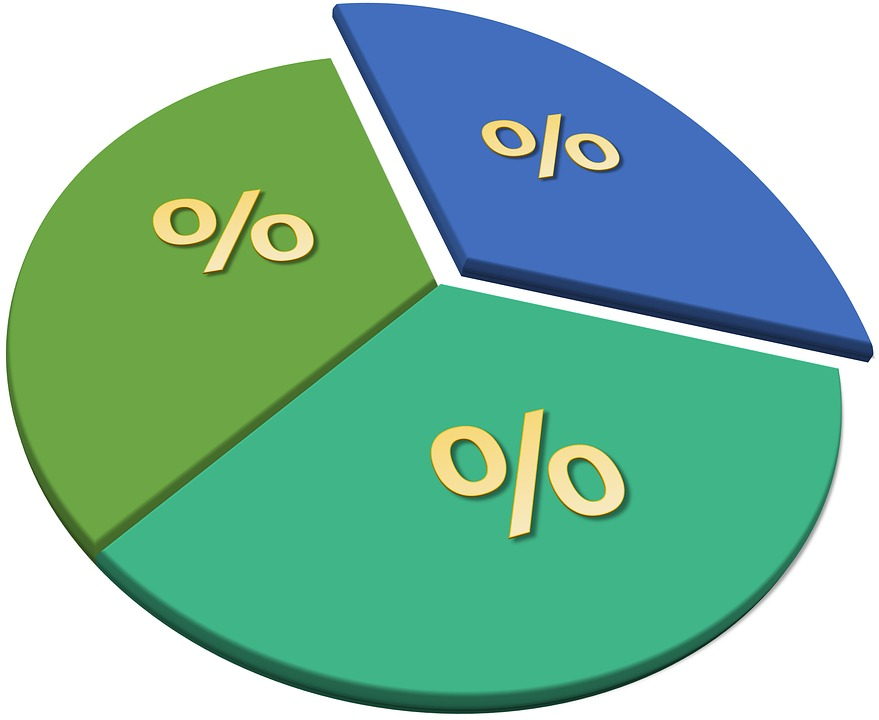> > How to Calculate Percentage

# How to Calculate Percentage

We often wonder how to calculate percentage and it does look tough. However, let us assure you that it is not as tough as it looks, in fact, it can be quite easy if you use the right technique. In today’s world where everything is online, we also have percentage calculators present online which is just a single search away. But, it is not always possible to rely on them as we need to calculate percentages without any assistance many a time. So, before learning how to calculate percentage, we must first understand what percentages are.

## Meaning of Percentage

The term ‘percentage’ is derived from the word per cent. When we split it into the root words, w get ‘per’ and ‘cent’. Cent is basically an old European word having French, Italian, Latin origins which means ‘hundred’. Thus, if you get 97 percent, it literally means 97 per 100. So, you see that if it has rained 14 times in the last 100 nights, it rained 14 percent of the time.### How to Calculate Percentage

Now, we see that when you get to convert percentages, the numbers you will get will be in two different formats. These formats are going to be either decimals or fractions. Moreover, we see that it is indeed easier to calculate percentage in decimal format. When you wish to convert a decimal into a percentage, you simply need to multiply it by 100. For instance, when we have to convert .95 to a percent, we will have to simply multiply .95 by 100.

.95 × 100 = 95.

Therefore, our result will be 95 per cent.

### Fraction into Percentage

If we get a fraction which we need to convert into a percentage, we need to simply divide the top number, which is our numerator, by the bottom number, which is our denominator. For instance, if the fraction is 15/100, we will have to divide 15 by 100.

15 ÷ 100 = .15

After that, we will follow the steps above in order to convert a decimal to a per cent.

.15 × 100 = 15

Therefore, our answer will be 15%.

### Other Methods of How to Calculate Percentage

All this was comparatively easy. The more complex task is when we are required to know a percentage when we get numbers that do not fit so precisely into 100. Most often, we will get a percentage of a given number.

For instance, you know that 20 per cent of your salary will go to taxes and you need to find out how much money will be 20 per cent of your salary. In order to calculate the percentage of a particular number, we need to start by converting the percentage number to a decimal.

In this way, we will have to take the reverse process of what we have done previously. So, begin by dividing the percentage by 100. Thus, 20% means 20 which we divide by 100 or .20.

20 ÷ 100 = .20

Once we have got the decimal version of our percentage, we will have to then multiply it by the given number. Over here, it is the amount of salary. If your salary is Rs. 15,000, you will have to multiply 15,000 by .20.

15,000 × .20 = 3,000

Thus, we have our answer as 3,000. So, you will be paying Rs. 3,000 in taxes.

### Example

You have to send 20 per cent of your salary for the next 5 months to pay for your sister’s tuition fees. If your salary is Rs. 20,000, how much will you have to save?

We will begin by converting 20 per cent to a decimal

20 ÷ 100 = .20

After that, multiply the decimal by the amount of your salary which is 20,000.

20,000 × .20 = 4,000

Therefore, you will have to save Rs. 4,000 from every salary.

## Solved Question for You

Question 1: What do you mean by percentage?

Answer: In math, a percentage is a number or ratio which we express as a fraction of 100. We make use of the sign % to denote percentage. It is derived from a Latin term ‘per centum’ which means by a hundred.

Share with friends

## Customize your course in 30 seconds

##### Which class are you in?
5th
6th
7th
8th
9th
10th
11th
12th
Get ready for all-new Live Classes!
Now learn Live with India's best teachers. Join courses with the best schedule and enjoy fun and interactive classes.Ashhar Firdausi
IIT Roorkee
BiologyDr. Nazma Shaik
VTU
ChemistryGaurav Tiwari
APJAKTU
Physics
Get Started

## Browse

##### Percentages0 Followers

Most reacted comment
1 Comment authorsRecent comment authors
Subscribe
Notify ofGuest
kiran

Total wrong teaching and examples are wrong change the mistakes of every example, I found this
change in percentage = 4/40 x 100 = 20%.

## Question Mark?

Have a doubt at 3 am? Our experts are available 24x7. Connect with a tutor instantly and get your concepts cleared in less than 3 steps.